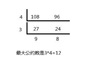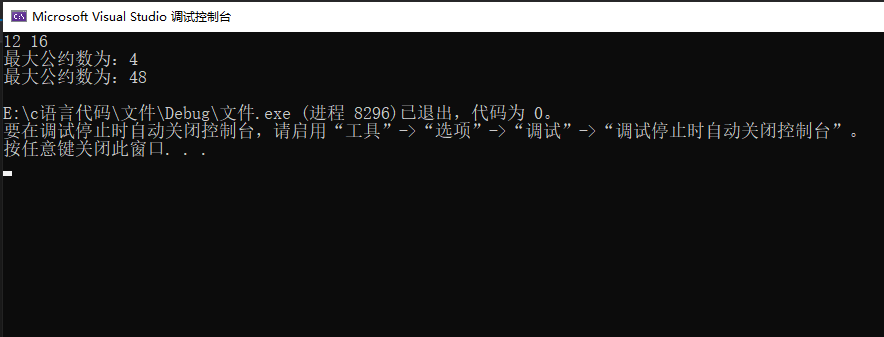Highest Common Factor(HCF)

Greatest Common Divisor(GCD)

• 问题：请从键盘上输入两个数值 x，y，请用C语言求出这两个数值的最大公约数与最小公倍数。 首先，我们要想解决这道问题，就要了解什么是最大公约数与最小公倍数。 最大公因数；也称最大公约数、最大公因子，指两个或...
C语言三种算法求解最大公约数与最小公倍数

最大公约数与最小公倍数的求解是很多初学C的人所面临的一道问题。当然这道问题并不难解答，也有很多人已经写过相关的博客，我在此书写此篇博客，一是为了让自己能够夯实基础，另外就是希望能够帮到和我一样的初学者。

当然，在写这篇博客之前，我已经做过相关资料的调查，可能读者会发现此篇博客会与其他人的博客有所重复，但是，我保证绝未抄袭。好了，进入正题！

问题：请从键盘上输入两个数值 x，y，请用C语言求出这两个数值的最大公约数与最小公倍数。

首先，我们要想解决这道问题，就要了解什么是最大公约数与最小公倍数。

最大公因数；也称最大公约数、最大公因子，指两个或多个整数共有约数中最大的一个。----来源百度百科

最小公倍数：两个或多个整数公有的倍数叫做它们的公倍数。----来源百度百科

了解了其含义，接下来就是构思算法，通常而言，求解最大公约数有三种算法，而最小公倍数的求解，我们可以很容易的推断出，最小公倍数等于两个数值的乘积除以这两个数值的最大公约数。那么接下来的算法我将在此一一进行列举和解释。

1.辗转相除法：

又名欧几里德算法（Euclidean algorithm），它是已知最古老的算法， 其可追溯至公元前300年前。 ----来源百度百科

辗转：望文生义，就是翻来覆去。相除就很好理解了，就是进行除法运算。

辗转相除法的核心就是不断的让两个数做除法运算。其原理基于两个整数的最大公约数等于其中较小的数和两数的相除余数的最大公约数。

假设两数为 x，y。

先令 z = x % y ;

之后 y 赋给 x 即令  x = y ;

再将 z 赋给 y 即令  y = z；

辗转相减，其终止条件为：y 等于0时。

代码如下：

#include<stdio.h>
int main()
{
int x, y, z, m, n;
printf("请输入两个数：");
scanf_s("%d%d", &x, &y);
m = x, n = y;
while (y != 0)
{
z = x%y;
x = y;
y = z;
}
printf("最大公约数是: %d\n", x);
printf("最小公倍数是: %d\n", m*n / x);
system("pause");
return 0;
}

2.辗转相减法：

即尼考曼彻斯法，其特色是做一系列减法，从而求得最大公约数。----来源百度百科

辗转相减法即通过对两数的不断减法运算。

假设两数为 x, y。

当 x > y 时，令 x = x - y;

反之，则令 y = y - x;

之后一直辗转相减，直至 x = y 时，终止。

代码如下：

#include<stdio.h>
int main()
{
int x, y, m, n;
printf("请输入两个数：");
scanf_s("%d%d", &x, &y);
m = x, n = y;
while (x!=y)
{
if (x>y)
x = x-y;
else
y = y-x;
}
printf("最大公约数是: %d\n", x);
printf("最小公倍数是: %d\n", m*n / x);
system("pause");
return 0;
}

3.穷举法：

穷举法的基本思想是根据题目的部分条件确定答案的大致范围，并在此范围内对所有可能的情况逐一验证，直到全部情况验证完毕。----来源百度百科

穷举法又称枚举法，通过对数值范围内的所有数字进行检验，得出其结果。

代码如下：

#include<stdio.h>
int main()
{
int x, y, i, m, n;
printf("请输入两个数：");
scanf_s("%d%d", &x, &y);
m = x, n = y;
for (i = x; i > 0; i--)
{
if (x%i == 0 && y%i == 0)
break;
}
printf("最大公约数是: %d\n", i);
printf("最小公倍数是: %d\n", m*n / i);
system("pause");
return 0;
}

以上即为求解最大公约数与最小公倍数的三种算法，如有纰漏，还请各位不吝赐教。


展开全文• 运行最大公约数的常用算法，并进行程序的调式与测试，要求程序设计风格良好，并添加异常处理模块（如输入非法等）。 二、算法 辗转相除法 辗转相除法（又名欧几里德法）C语言中用于计算两个正整数a,b的最大公约数和...
一、题目
运行最大公约数的常用算法，并进行程序的调式与测试，要求程序设计风格良好，并添加异常处理模块（如输入非法等）。
二、算法
辗转相除法

辗转相除法（又名欧几里德法）C语言中用于计算两个正整数a,b的最大公约数和最小公倍数，实质它依赖于下面的定理：
在这里插入图片描述
根据这一定理可以采用函数嵌套调用和递归调用形式进行求两个数的最大公约数和最小公倍数,现分别叙述如下

三、源代码
#include<stdio.h>

int main()
{
int x = 0;
int y = 0;
int z = 0;
scanf_s("%d%d", &x, &y);
z = x * y;

if (x < y)
{
int temp = x;
x = y;
y = temp;
}
int k = x % y;
while (k)
{
x = y;
y = k;
k = x % y;
}
printf("最大公约数为：%d\n", y);
printf("最大公约数为：%d\n", z/y);
return 0;
}

四、运行结果展开全文• ## 求最大公约数（辗转相除法）

万次阅读 多人点赞 2019-06-03 16:20:50
也称最大公因数、最大公因子，a， b的最大公约数记为（a，b），同样的，a，b，c的最大公约数记为（a，b，c），多个 整数的最大公约数也有同样的记号。求最大公约数有多种 方法，常见的有质因数分解法、短除法、辗转...

最大公约数（Greatest Common Divisor）指两个或多个整数共有约数中最大的一个。

也称最大公因数、最大公因子，a， b的最大公约数记为（a，b），同样的，a，b，c的最大 公约数记为（a，b，c），多个 整数的最大公约数也有同样的记号。求最大公约数有多种 方法，常见的有 质因数分解法、 短除法、 辗转相除法、 更相减损法。与最大公约数相对应的概念是 最小公倍数，a，b的 最小公倍数记为[a，b]。

再来介绍一下辗转相除法：

辗转相除法又叫欧几里得算法,是欧几里得最先提出来的.辗转相除法的实现,是基于下面的原理(在这里用(a,b)表示a和b的最大公因数)：
(a,b)=(a,ka+b),其中a、b、k都为自然数.………………①
也就是说,两个数的最大公约数,将其中一个数加到另一个数上,得到的新数,其公约数不变,比如(4,6)=(4+6,6)=(4,6+2×4)=2.要证明这个原理很容易：如果p是a和ka+b的公约数,p整除a,也能整除ka+b.那么就必定要整除b,所以p又是a和b的公约数,从而证明他们的最大公约数也是相等的.
基于上面的原理,就能实现我们的迭代相减法：
(78,14)=(64,14)=(50,14)=(36,14)=(22,14)=(8,14)=(8,6)=(2,6)=(2,4)=(2,2)=(0,2)=2
基本上思路就是大数减去小数,一直减到能算出来为止,在作为练习的时候,往往进行到某一步就已经可以看出得值.迭代相减法简单,不过步数比较多,实际上我们可以看到,在上面的过程中,由(78,14)到(8,14)完全可以一步到位,因为(78,14)=(14×5+8,14)=(8,14),由此就诞生出我们的辗转相除法.
用辗转相除法求(a,b).设r0=b,r1=a,反复运用除法算式,得到一系列整数qi,ri和下面的方程：
相当于每一步都运用原理①把数字进行缩小,上面右边就是每一步对应的缩小结果,可以看出,最后的余数rn就是a和b的公约数.迭代相减法和辗转相除法在本质上是一样的,相对来说,减法比较简单（需要10步）,但是除法步数少（仅需4步）.

因此可以通过这个原理来求出最大公约数：

#include<iostream>
#include<cstdio>
using namespace std;

int fun(int m,int n){
int rem;			//余数，当余数为0的时候，最后的m即为最大公约数
//先用较小的数对较大的数取余，再用余数对较小的数求余，直到余数为零
while(n > 0){
rem = m % n;
m = n;
n = rem;
}
return m;			//将结果返回
}
int main(){
int n,m;
cin>>m>>n;
cout<<"m和n的最大公约数为："<<fun(m,n)<<endl;
return 0;
} 

因为到余数为零结束，所以还可以将程序简化一下用递归来求：

int fun(int m,int n){
if(n==0) return m;
return fun(n,m%n);
}

再简化一下，用一行代码来求：

int gcd(int m, int n) {
return n ? gcd(n, m % n) : m;
}


展开全文辗转相除法
• 分享一个大牛的人工智能教程。... * 求两个自然数的最大公约数 - C++ - by Chimomo * * Answer：辗转相除法 */ #include &lt;iostream&gt; #include &lt;cassert&gt; #include &...
分享一个大牛的人工智能教程。零基础！通俗易懂！风趣幽默！希望你也加入到人工智能的队伍中来！请点击http://www.captainbed.net

/*
* 求两个自然数的最大公约数 - C++ - by Chimomo
*
* Answer：辗转相除法
*/

#include <iostream>
#include <cassert>
#include <stack>
#include <math.h>

using namespace std;

int GreatestCommonDivisor(int a, int b) {
int t;

if (a < b) {
// 交换两个数，使大数放在a的位置上。
t = a;
a = b;
b = t;
}

while (b != 0) {
// 利用辗转相除法，直到b为0为止。
t = a % b;
a = b;
b = t;
}

return a;
}

int main() {
cout << GreatestCommonDivisor(318, 87632) << endl;
return 0;
}

// Output:
/*
2

*/



展开全文算法 C++
• 先求 最大公约数 （辗转相除法．）： 以小数除大数，如果能整除，那么小数就是所求的最大公约数．否则就用余数来除刚才的除数；再用这新除法的余数去除刚才的余数．依此类推，直到一个除法能够整除，这时作为除数的...算法...